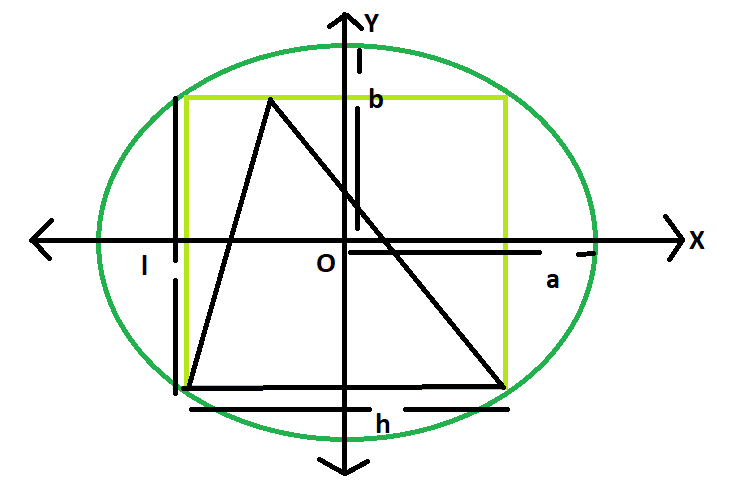Area of a triangle inscribed in a rectangle which is inscribed in an ellipse

• Last Updated : 15 Mar, 2021

Given here is an ellipse with axes length 2a and 2b, which inscribes a rectangle of length l and breadth h, which in turn inscribes a triangle.The task is to find the area of this triangle.
Examples:

Input: a = 4, b = 3
Output: 12

Input: a = 5, b = 2
Output: 10Approach
We know the Area of the rectangle inscribed within the ellipse is, Ar = 2ab(Please refer here),
also the area of the triangle inscribed within the rectangle s, A = Ar/2 = ab(Please refer here)
Below is the implementation of the above approach:

C++

 // C++ Program to find the area of the triangle// inscribed within the rectangle which in turn// is inscribed in an ellipse#include using namespace std; // Function to find the area of the trianglefloat area(float a, float b){     // length of a and b cannot be negative    if (a < 0 || b < 0)        return -1;     // area of the triangle    float A = a * b;    return A;} // Driver codeint main(){    float a = 5, b = 2;    cout << area(a, b) << endl;    return 0;}

Java

 // Java Program to find the area of the triangle// inscribed within the rectangle which in turn// is inscribed in an ellipse import java.io.*; class GFG { // Function to find the area of the trianglestatic float area(float a, float b){     // length of a and b cannot be negative    if (a < 0 || b < 0)        return -1;     // area of the triangle    float A = a * b;    return A;} // Driver code      public static void main (String[] args) {    float a = 5, b = 2;    System.out.println(area(a, b));    }}//This code is contributed by anuj_67..

Python3

 # Python 3 Program to find the# area of the triangle inscribed# within the rectangle which in# turn is inscribed in an ellipse # Function to find the area# of the triangledef area(a, b):         # length of a and b cannot    # be negative    if (a < 0 or b < 0):        return -1     # area of the triangle    A = a * b    return A # Driver codeif __name__ == '__main__':    a = 5    b = 2    print(area(a, b))     # This code is contributed# by Surendra_Gangwar

C#

 // C# Program to find the area of// the triangle inscribed within// the rectangle which in turn// is inscribed in an ellipseusing System; class GFG{     // Function to find the// area of the trianglestatic float area(float a, float b){     // length of a and b    // cannot be negative    if (a < 0 || b < 0)        return -1;     // area of the triangle    float A = a * b;    return A;} // Driver codestatic public void Main (){    float a = 5, b = 2;    Console.WriteLine(area(a, b));}} // This code is contributed by ajit



Javascript


Output:
10

My Personal Notes arrow_drop_up